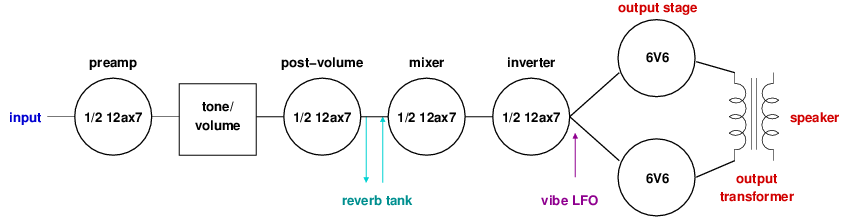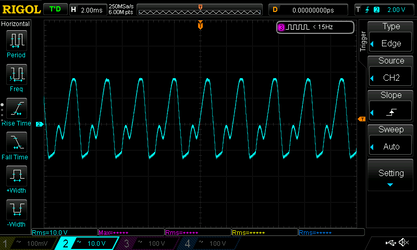# Mojotone Princeton Reverb Clone Project

## Audio signal trace

On this page we trace the path of the audio signal through the amp. Here is a high-level block diagram of the amp's audio signal path:Audio signals enter the amp through one of the two input jacks and are directly connected to the grid input of one of the triodes in the 12ax7 (or 7025) preamp tube. The main difference between the two input jacks is the resistors: channel one has a higher resistance to ground so more of the audio signal makes its way into the amp. After exiting the preamp, the audio signal then goes through the tone stack and then the volume potentiometer. The passive tone stack reduces the level of the audio signal, so the output of the volume pot is sent to the post-volume 12ax7 triode to reamplify it. The output of the post-volume 12ax7 tube forks off a path to the reverb circuit and then returns to join with the original signal on the input of the mixer 12ax7 triode. The output of the mixer 12ax7 triode is then fed into the input of the inverter 12ax7 triode. The inverter tube outputs two audio signals where one signal is out of phase with the other. Out of phase audio signals are used to drive the output tubes of a push-pull style amplifier like the Princeton. The low-frequency oscillator output of the vibe circuit is also fed into the inputs of the output tubes to modulate the volume. Finally, the output of the 6V6 power tubes is fed into the primary side of the output transformer to convert the high-voltage low-current output of the 6V6's into a low-voltage high-current signal that is suitable for driving the amp's speaker.

To trace the audio path, I used an audio signal generator generating a sine wave as input to the amplifier. Instead of a speaker, I connected a dummy load to the amp output so that I would not have to listen to a sine wave while conducting tests.

### Preamp

The first figure shows the input from the signal generator in yellow and the output of the preamp triode in blue. Note that the signals are on different scales with the yellow on 100mV per block and the blue on 5V per block, so the yellow input signal is 119mV RMS and the blue output signal is 6.01V RMS. So the preamp triode significantly amplifies the input signal as expected. It also inverts the phase of the input signal.

The second figure adds a pink plot that shows the signal as it enters the volume pot after exiting the tone stack. The treble and bass potentiometers are both set to 5. Passing through the passive tone stack has reduced the signal down to 668mV RMS.

### Tone stack

The next four plots show the same three signals as the previous plot with different tone settings on the treble and bass pots. The settings are: treble 1/bass 5, treble 10/bass 5, treble 5/bass 1, treble 5/bass 10. The plots clearly show that changing the tone knobs impacts both the amplitude and phase of the signal coming out of the tone stack.

### Post-volume output

On the next plot we move a probe so that the pink signal is now the output of the post-volume triode. I adjusted the volume knob so that the post-volume triode output level matches the input level to the tone stack. To achieve this the volume knob needs to be set at about 6.5.

Using this configuration, I also made a table recording volume knob settings and post-volume triode output voltages. From the table you can see the logarithmic taper used on the volume pot. It also shows volume levels 1 and 2 have about the same output.

Volume settingOutput (volts) Volume settingOutput (volts)
10.15 64.88
20.15 77.83
31.16 816.8
42.49 924.5
53.71 1033.8

### Mixer

The next figure shows the output of the post-volume triode in yellow, the input to the mixer triode in blue, and the output of the mixer triode in pink. The difference between the yellow and blue curves is due to the reverb tank circuit. The yellow curve is 6.22 V RMS while the blue curve is 746 mV RMS. The pink curve shows that mixer triode increases the signal back up to 25.7 V RMS.

### Inverter

The next two plots show the output of the mixer in yellow, the input to the inverter in blue, and the plate output of the inverter in pink. The first plot is with the volume set to 4.5 and the second is with the volume set to 6.5 where the signal starts to distort some for the current input.

The next figure is with the volume set to 5, and it shows the input to the inverter in yellow and the two outputs of the inverter in blue and pink. The input voltage is 15.9 V RMS. The output voltages are 14.6 V RMS (blue, plate) and 14.9 V RMS (pink, cathode). This shows that the inverter does not really amplify the signal much, it just inverts it on one of the outputs.

### Output stage

The next plot shows the input and output signals of the 6V6 power tubes. The yellow and blue plots are the two input signals and they are 14.7 V RMS and 15.0 V RMS respectively. The pink and dark blue plots show the outputs of the power tubes. In this case both output curves are 142 V RMS.

### Output transformer

The next plot shows the inputs and outputs of the output transformer. The yellow plot shows the 8.29 V RMS output signal of the output transformer. The pink 144 V RMS and dark blue 145 V RMS plots show the two inputs to the output transformer. The output transformer takes a high-voltage low current signal as input and generates a low-voltage high current signal as output to drive the speaker.

### Vibe LFO

The next two plots show the LFO generated by the oscillator in the vibe circuit. The first plot shows the LFO with the speed knob set to 1, while the second plot shows the LFO with the speed knob set to 10. The horizontal scale of these plots is 50msec per block. The cycle time of the LFO with speed 1 is about 275 msec, while the cycle of the LFO with speed 10 is about 100 msec.

Here is a table with LFO cycle times for a full range of speed knob settings.

Speed settingCycle time (msec) Speed settingCycle time (msec)
1275 6138
2263 7125
3225 8113
4175 9100
5150 10100

### Input to speaker

The next four plots show the overall input to output signal with various volume settings. The yellow plot in the first figure shows the input signal which is 115 mV RMS. The next three plots show the output signal at volume levels 6, 8, and 10 respectively. These plots show how the input signal distorts at higher volume levels.

### Reverb

The next two plots show the speaker output using the same yellow input signal as show above. We set the volume to 6 to keep the signal from being distorted. The first plot shows the output signal with the reverb set to 5. The second plot shows the output signal with the reverb set all the way up to 10.